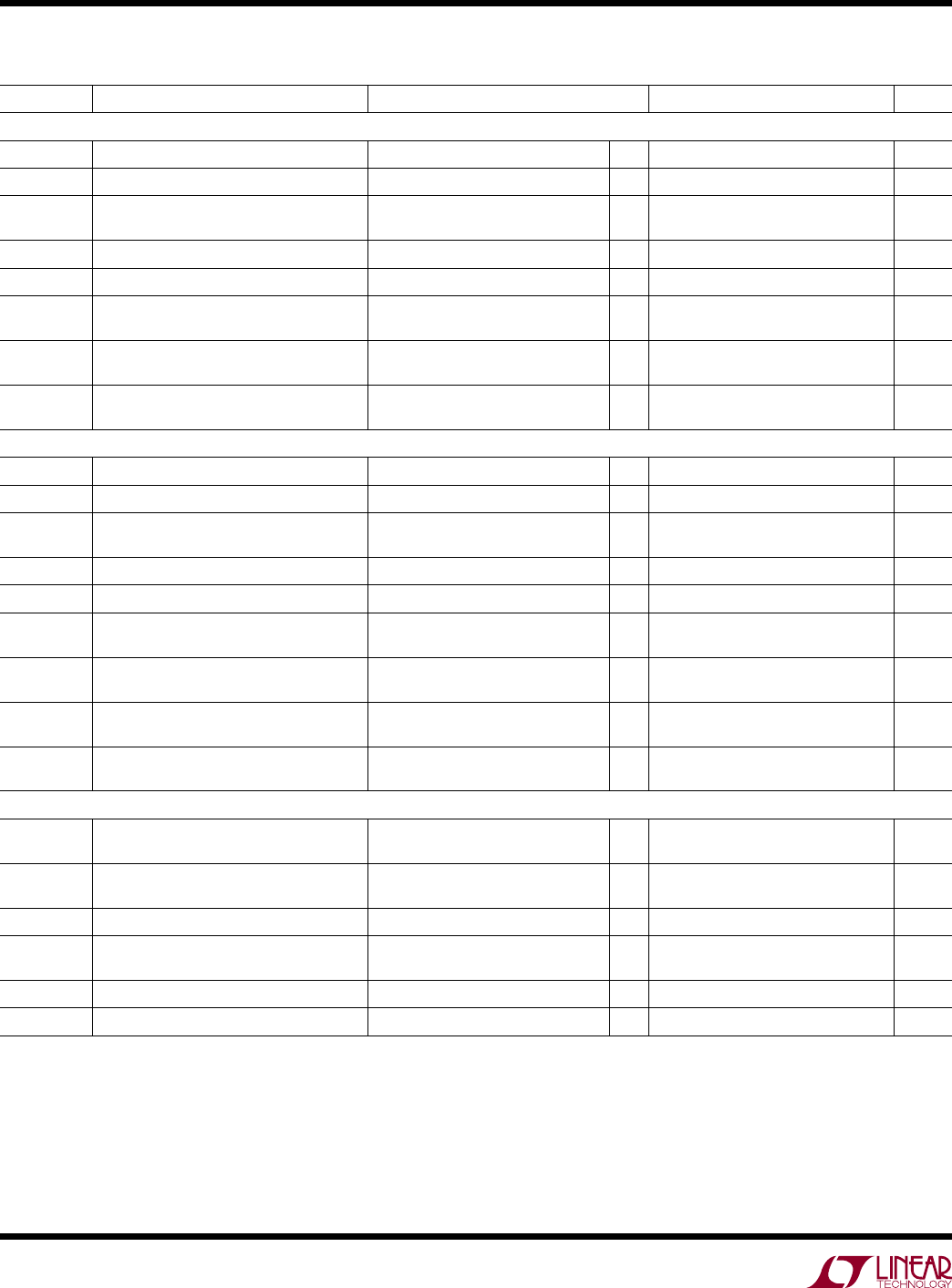# DatasheetLTC2862/LTC2863/
LTC2864/LTC2865
6
2862345fc
SYMBOL PARAMETER CONDITIONS MIN TYP MAX UNITS
Driver – High Speed (LTC2862-1, LTC2863-1, LTC2864-1, LTC2865 with SLO High)
f
MAX
Maximum Data Rate (Note 3)
l
20 Mbps
t
PLHD
, t
PHLD
Driver Input to Output R
DIFF
= 54Ω, C
L
= 100pF (Figure 4)
l
25 50 ns
Δt
PD
Driver Input to Output Difference
|t
PLHD
– t
PHLD
|
R
DIFF
= 54Ω, C
L
= 100pF (Figure 4)
l
2 9 ns
t
SKEWD
Driver Output Y to Output Z R
DIFF
= 54Ω, C
L
= 100pF (Figure 4)
l
±10 ns
t
RD
, t
FD
Driver Rise or Fall Time R
DIFF
= 54Ω, C
L
= 100pF (Figure 4)
l
4 15 ns
t
ZLD
, t
ZHD
,
t
LZD
, t
HZD
Driver Enable or Disable Time R
L
= 500Ω, C
L
= 50pF, RE = 0V
(Figure 5)
l
180 ns
t
ZHSD
, t
ZLSD
Driver Enable from Shutdown R
L
=500Ω, C
L
= 50pF, RE = High
(Figure 5)
l
9 µs
t
SHDND
Time to Shutdown R
L
= 500Ω, C
L
= 50pF, RE = High
(Figure 5)
l
180 ns
Driver – Slew Rate Limited ( LTC2862-2, LTC2863-2, LTC2864-2, LTC2865 with SLO Low)
f
MAX
Maximum Data Rate (Note 3)
l
250 kbps
t
PLHD
, t
PHLD
Driver Input to Output R
DIFF
= 54Ω, C
L
= 100pF (Figure 4)
l
850 1500 ns
Δt
PD
Driver Input to Output Difference
|t
PLHD
– t
PHLD
|
R
DIFF
= 54Ω, C
L
= 100pF (Figure 4)
l
50 500 ns
t
SKEWD
Driver Output Y to Output Z R
DIFF
= 54Ω, C
L
= 100pF (Figure 4)
l
±500 ns
t
RD
, t
FD
Driver Rise or Fall Time R
DIFF
= 54Ω, C
L
=100pF (Figure 4)
l
500 800 1200 ns
t
ZLD
, t
ZHD
Driver Enable Time R
L
= 500Ω, C
L
= 50pF, RE = 0V
(Figure 5)
l
1200 ns
t
LZD
, t
HZD
Driver Disable Time R
L
= 500Ω, C
L
= 50pF, RE = 0V
(Figure 5)
l
180 ns
t
ZHSD
, t
ZLSD
Driver Enable from Shutdown R
L
= 500Ω, C
L
= 50pF, RE = High
(Figure 5)
l
10 µs
t
SHDND
Time to Shutdown R
L
=500Ω, C
L
= 50pF, RE = High
(Figure 5)
l
180 ns
t
PLHR
, t
PHLR
L
= 15pF, V
CM
= 1.5V, |V
AB
| = 1.5V,
t
R
and t
F
< 4ns (Figure 6)
l
50 65 ns
t
SKEWR
|t
PLHR
– t
PHLR
|
C
L
= 15pF (Figure 6) 2 9 ns
t
RR
, t
FR
Receiver Output Rise or Fall Time C
L
= 15pF (Figure 6)
l
3 12.5 ns
t
ZLR
, t
ZHR
,
t
LZR
, t
HZR
L
= 1k, C
L
= 15pF, DE = High (Figure 7)
l
40 ns
t
ZHSR
, t
ZLSR
L
= 1k, C
L
= 15pF, DE = 0V, (Figure 7)
l
9 µs
t
SHDNR
Time to Shutdown R
L
= 1k, C
L
= 15pF, DE = 0V, (Figure 7)
l
100 ns
swiTchinG characTerisTics
The l denotes the specifications which apply over the full operating
temperature range, otherwise specifications are at T
A
= 25°C. V
CC
= V
L
= 3.3V unless otherwise noted. (Note 2)
Note 1. Stresses beyond those listed under Absolute Maximum Ratings
may cause permanent damage to the device. Exposure to any Absolute
Maximum Rating condition for extended periods may affect device